#Function Repository Resource:

Give the adjugate matrix of a square matrix

Contributed by: Daniel Lichtblau
 ResourceFunction["Adjugate"][mat] gives the transpose of the cofactor matrix of mat.

## Details and Options

The matrix product of a matrix m with its adjugate is diagonal, with all diagonal entries equal to the determinant of m.

## Examples

### Basic Examples

Form the adjugate of a matrix of dimension 2:

 In:=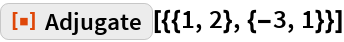Out=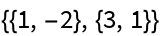### Scope

The adjugate is a square matrix of the same dimension:

 In:=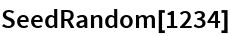In:=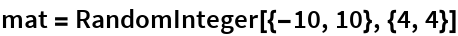Out=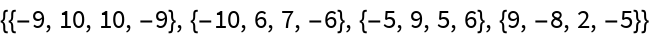In:=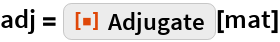Out=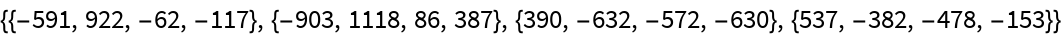The product of the adjugate with the matrix is a diagonal matrix with the same values on the diagonal:

 In:=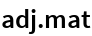Out=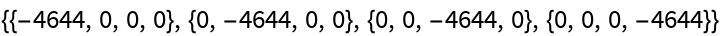The values on the diagonal are the determinant of the matrix:

 In:=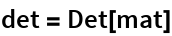Out=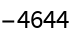When a matrix is invertible, the adjugate divided by the determinant gives the inverse:

 In:=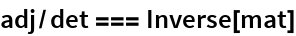Out=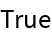The adjugate is defined for symbolic as well as numeric matrices:

 In:=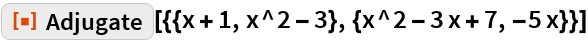Out=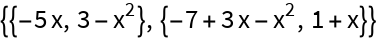A square matrix need not be invertible:

 In:=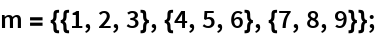This matrix is not invertible because it does not have full rank:

 In:=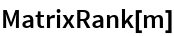Out=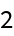The adjugate is defined even when the inverse does not exist:

 In:=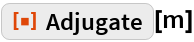Out=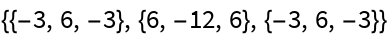### Properties and Relations

The adjugate satisfies a simple identity:

 In:=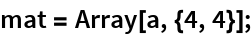In:=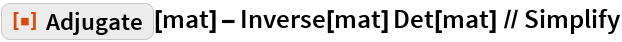Out=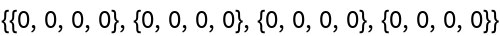The adjugate and the matrix of minors are the same up to reordering and signs:

 In:=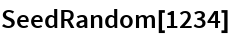In:=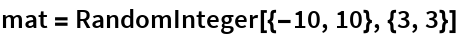Out=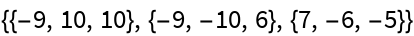In:=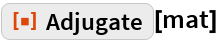Out=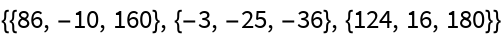In:=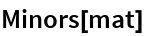Out=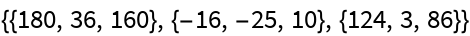## Requirements

Wolfram Language 11.3 (March 2018) or above